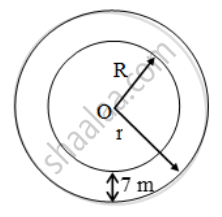# The inner circumference of a circular track is 220 m. The track is 7m wide everywhere. Calculate the cost of putting up a fence along the outer circle at the rate of j – 2 per metre. - Mathematics

Sum

The inner circumference of a circular track is 220 m. The track is 7m wide everywhere. Calculate the cost of putting up a fence along the outer circle at the rate of j – 2 per metre. (Use π = 22/7)

#### Solution

Let the inner and outer radii of the circular track be r metres and R metres respectively. Then, Inner circumference = 220 metres⇒ 2πr = 220 ⇒ 2 × \frac { 22 }{ 7 } × r = 220 ⇒ r = 35 m

Since the track is 7 metre wide everywhere. Therefore,

R = Outer radius = r + 7 = (35 + 7) m = 42 m

∴

Rate of fencing = j – 2 per metre

∴ Total cost of fencing

= (Circumference × Rate) = j – (264 × 2) = j – 528

Concept: Circumference of a Circle
Is there an error in this question or solution?